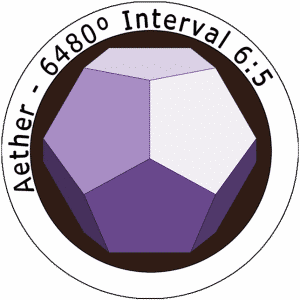In part 3 we’ll explore how esoteric number theory illuminates the primordial interiors of the universe. In the spirit of Pythagoreanism, we use arithmetic and geometry to identify features of reality’s formative level, showing how emptiness gives rise to form and issues forth order from chaos.

(adapted from the forthcoming book Integral Esoterics by Asa)

For every inside there is an outside, and for every outside there is an inside; though they are different, they go together. —Alan Watts, Man, Nature, and the Nature of Man, 1991

In part 2 we began exploring the interior nature of triangles. We briefly explored how 3-ness plays a role in bringing order from chaos. In this section we will look even deeper into some of the underlying patterns of nature to explore how esoteric number theory illuminates the primordial interiors as it draws forth definiteness from a resplendent undifferentiated totality. In the spirit of Pythagoreanism we will use arithmetic and geometry to identify some key features of the formative level of reality where the numinous can be seen encoding natures algorithms. If you haven’t read parts 1 & 2, you’ll want to do that before continuing. pt 1, pt 2

~*~

Pythagorus of Samos, who was – it would seem – a Panpsychist, founded the Pythagorean movement and significantly influenced Greek thought. Plato, Aristotle, and later Nicholas Copernicus, Joannes Kepler and Issac Newton where known to have been deeply influenced by Pythagoreanism. He gave us the earliest recorded mathematical system for music, decoded triangles with the pythagorean theorem, contributed to astronomy, invigorated the idea of reincarnation in the west, and gave us what we now call numerology. There are many ideas attributed to Pythagorus which may or may not have come from him. This is because he had a number of followers that made contributions to the ideas of Pythagoreanism long after he died. Perhaps one of the most significant contributions he left us was the philosophical super-food, Pansychism – a nutrient of understanding western thought still has yet to fully bring into its diet.

Panpsychism /panˈsʌɪkɪz(ə)m/ : In philosophy, panpsychism is the view that consciousness, mind, or soul (psyche) is a universal and primordial feature of all things. Panpsychists see themselves as minds in a world of mind.

The Pythagoreans where probably vegetarian, practiced meditation, were devoted to athletics, and used music, art and math as a kind of spiritual practice. At the heart of the Pythagorean philosophy is a unitive cosmology that holds numbers and their arithmetic and geometric properties to be a part of a natural, divine law that can be decoded by careful observation. For Pythagoreans an exploration of the fundamental constituents of measure and form brings us closer to transcendental and universal truths that can build virtue in our souls by attending to their depths.

This idea lives on today being elaborated on through several glorious lineages. In addition to Kabbalah and Qabalah, Alchemy, Theosophy, Neo-Pythagoreanism and Neo-Platonism in the west, we also have the rich lineages of Jain and Vedic philosophy and mathematics in the east. There are many fruit baring branches on the tree of life. Those who’s legacies have persisted through time, have done so largely because the teachings on ageless wisdom are fully enshrined in the seeds of mathematics and geometry. Storing and distributing seeds of esotericism means that their perennial wisdom will always be available for the next generation of gardeners.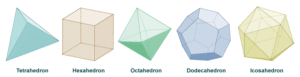### From Seeds to Flowers to Fruits

We learn through Plato, who was also influenced by Pythagorus that there are 5 fundamental shapes that make up all the forms in nature and that these shapes exists as eternal ideas out side of time. Each of these shapes are nested, one inside the other in a way that makes what’s called Metatron’s cube. The classical elements are the basic constituents of reality and are each themselves a nested holarchy of both facts and values coexisting, but they’re ensconced in a shell which must be decomposed for the germ to begin to unfurl into foliage. The four classical elements and the fifth their synthesis, are unambiguously displaying their mathematical facts. But to get at their more interior components – the values or meanings they point to, is a process of gardening.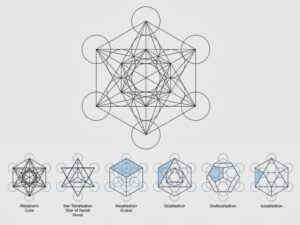To germinate these forms is useful and perhaps even essential for an understanding of the mytho-poetic narratives of panpsychism. Sacred geometry tells the story of how something comes from nothing, what mind is and what evolution is up to. More generally, the study of math and geometry in the light of the perennial grand narrative it inevitably implies, cultivates a capacity for synthesis thinking. That is to say, it brings about an adeptship of a type of logic that has at its disposal some of the tools and insights of other domains of knowledge. Chemistry, biology, physics, philosophy and metaphysics need not be disparate fields. They are, according to the panpsychist view, different forms of the expression of one thing – namely mind or Spirit. This one thing, whatever we choose to call it, is of such exquisite beauty and goodness that its observers are taken beyond the boundaries of an apparent disunity into a perception of pure divinity. This Alethea, being both ecstatic and pragmatic mutates cognitive processes, re-organizing perception into a holarchal super-symmetry or over-unity that pervades everything.

As a kind of meditation, esoteric number theory brings about a reversal of our solipsism (egoic self contraction), taking us out of ourselves and into the larger field of knowing, where the numinous can be clothed and its bare splendor made opaque. The mystery schools have pointed out in a number of ways, that a view of the numinous requires a means to clothe transparent streams of heavenly scintillation in to visible artifacts. Specific forms or objects are needed so that the eye of the body, and the eye of the mind can examine them there by bring about an opening of the lid of the eye of spirit.

With these mental objects to focus on, the formative level of reality can be perceived. Without them, the ineffable radiance of Spirit slips through our fingers as disembodied abstraction. Objects and subjects, facts and values, the relative and the absolute remain disconnected from each other and from a felt sense of gnostic apprehension.

The mathematical meditations like those performed in the Pythagorean mystery schools serve to provide us with objects of focus and grant us access to a view of a higher order underlying the very fabric of mind and matter. But this capacity is not a product of the method as much as it is an inherent feature of the observer and the observed. There’s no metaphysical hocus pocus here. Having a look at the anatomy that undergurds the structure of reality is a matter of attentiveness more than anything. Should desire be calling for such a self immolating thrill of revelation, a seeker need only practice a handful of basic skills.Plato’s simplest shape, the tetrahedron, is four equilateral triangles. The tetrahedron is the simplest regular 3d polygon. Its four vertices connecting its 4 equilateral triangles can be said to sum up the foundational structure of creation. In 2d, the triangle is the first shape that can be made. With only two lines there is no possibility of a 2d plane with a perimeter. But with three lines we have our first complete plane.Built into that plane automatically, is the potential for 4 other triangles to exists inside of it such that there will be a ratio of 4 : 1 between the smaller triangles to the larger whole. This feature establishes a harmonic proportion between the first form and all its subsequent iterations. This is a significant factor we’ll explore more deeply when looking at the neuro-harmonics of the four primary state stages. We then take the 1 : 4 triangle from 2d into 3d to make the tetrahedron.

A famous mathematical object that helps us explore the properties of the triangle and the tetrahedron is called the Tetractys. This form was a central idea in the Pythagorean school and is used as a teaching device in the western mystery schools still today. Its also the basis for harmonics in music, the basis for time keeping, and as I will attempt to demonstrate later on the organizational pattern that conjoins vertical and horizontal types of complexity.

So let’s explore the tetractys in depth, with reference to a few of its correlations to gematria, in an attempt to find out what harmony is, where coherence comes from, and how we might come to think of reality in a more comprehensive way. Along the way we will encounter the origin of the Hebrew alphabet, the magnificent 3 6 9, getting our feet wet in the field that is appropriately called the technology of the abyss.

In ordinary math triangle numbers count objects arranged in an equilateral triangle. The nth triangular number is the number of dots in the triangular arrangement with n dots on a side, and is equal to the sum of the n natural numbers from 1 to n.

The Pythagoreans call this the tetractys, or tetrad, or the tetractys of the decad (meaning the 4 of 10). In this triangular figure consisting of ten points arranged in four rows: one, two, three, and four points in each row consecutively, is the geometrical representation of the fourth triangular number. As a mystical symbol, this shape is the smallest representation of creation that remains complete.

You’ll notice that in the tetractys there are 9 small triangles, 3 larger triangles (overlapping). You’ll also notice, that it takes exactly 6 triangles, edge to edge, to complete a rotation. If we add up all the angles in the tetractys we get 1620 degrees, which you’ve probably already guessed reduces to 9. But let’s focus on its 4-ness.

When Pythagoreans and Kabbalists think of fours, a humble reverence fills the air. There are four seasons, four cardinal directions and four irreducible domains of reality – internal, external, individual and multiple. Matter, body, mind and spirit. Mineral, plant, animal and human. In physics its the strong force, the weak force, gravity and electromagnetism. In Kabbalah the four worlds are Asssiah, Yetzirah, Beriah and Atziluth or Action, Formation, Creation and Emanation, or in the language of integral philosophy there are the four state stages: Gross, Subtle, Causal and Non-dual.

Another instance of the tetrad in nature is in the elements carbon, hydrogen, nitrogen and oxygen. A theory in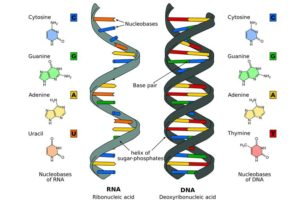evolutionary biology suggests that the genesis of life, complex amino acids were assembled by a strand of RNA which is made up of adenine, cytosine, guanine and uracil. A,C,D & U are themselves assembled from carbon, hydrogen, nitrogen and oxygen.

DNA molecules made from A, G, C, T are base pairs of the arrangement of C, H, O, N atoms. In the domains of chemistry, biology, physics, and mathematics, the quaternity or tetrad is instantiated rather clearly. The reason for this we will see, has its root in number theory and the recursion principal inherent in triangles.

Carl Jung elaborated extensively on the quaternio in his series of books called “Aion”. In Aion he explores the significance of the Allegoria Christi the symbol of Christ in relationship to the development and assimilation of the psychic totality of the self; The ego, the shadow, and animus and the anima. In it he draws from Gnostic and Alchemical symbolism to explore the problem of duality in relationship to our psychological developmental evolution. Together with the conscious, the unconscious and superconscious minds, the ego, the shadow the animus and the anima make up the triad in the tetrad of self, each aspect coming together to work out the condition of dualism.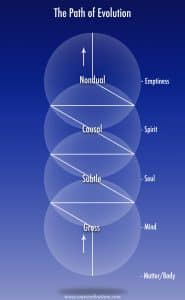The Kabbalists have elaborated extensively on the tetragrammaton, the four letter name of God. Y H V H or Jahweh or Jahova is not just a symbol for God as described by a mere tool of language. Its the structure of space-time itself, the fundamental constituent of reality, the underlying geometry and universal template for everything that ever was or ever will be. The name itself as its written, was build from the mathematical structure of the cosmos as a deep investigation of this science reveals. Later, we’ll see that the Chaldean Hebrew language was not created but discovered, and so in this case the word YHVH is also the thing it describes.

The Pythagoreans were deeply involved in the study of the four in the three. They submitted that the universe as expressed as the musica universalis – music of the spheres – which is made up of:

1. A Unity (Monad) – the basis of the Self
2. A Duality (Dyad) – the basis of Power
3. A Trinity (Triad) – the basis of Harmony
4. A Tetrad – The basis of the Kosmos

In the Tetractys, the four rows of dots add up to ten, which shows us a unity of a higher order (The Decad). The Tetractys then symbolizes the four classical elements—fire, air, water, and earth, from which all things are formed – symbolically. The Tetractys represents the simplest organization of existence that can be made in 2 dimensions. (its actually a 3d vector grid) The first row represents zero dimensions (a point). The second row represents one dimension (a line of two points). The third row represents three dimensions (a plane defined by a triangle of three points). The fourth row represents four dimensions (a tetrahedron defined by four points).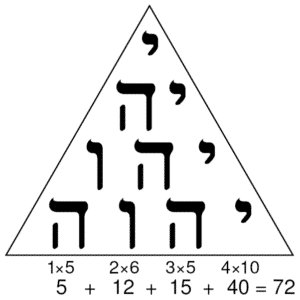In Kabalah the tetractys is shown using the tetragrammaton. This image suggests that God created the universe through the utterance of the name. Our definition of God gets profoundly updated by a study of this geometry. The tetragrammaton or the tetra-arising quality of being is significant. It provides a framework in which all the manifest universe arises.

Exploring the tetractys numerically, we see that when we add up the sum of digits 1 – 10 some of its esoteric underpinnings are made more obvious. In the tradition of the Pythagoreans, we’ll also use the digital root to get at an underlying simplicity. A digital root is the single integer, non – negative sum of a value obtained by an iterative process of summing digits. The process continues until a single-digit number is reached.

Why do we do this? Because we are looking for the irreducible component which will give us an expression of the simplest underlying pattern. This way we don’t get bogged down in too much complexity. Think of it like composting, or digesting a meal. In order to get the available nutrients out of a substance, we need to break it down to its smallest units. In the esoteric philosophy of numbers there are only 9 digits 1-2-3-4-5-6-7-8-9. 0 is everywhere an nowhere, like a fool, gallivanting through the dreams of the other numbers.

Here is the tetractys factored out:

1=1
1+1= 2
1+2 = 3
1+2+3 = 6
1+2+3+4 = 10

### The Tetrahedron is the Basis for the Ten Sephirah of the Tree of Life.

Our shape ends here, but in reality it can go on indefinitely. 4 though is a kind of minor completion because its a harmonically congruent expression. If we add to it we’d need to add a very specific number to maintain its harmony. Four rows of 10 dots is the smallest number we can use to have a complete notion or cycle of numbers. In just 4 numerals 10 is already present in latent form. 3 rows of 6 is too small a number for a complete notion, but with 10 we have the decimal system which provides the necessary means to maintain coherence even at higher levels of complexity.4 is the base. In 4 we have the square that is the base of the 6 sides that will become the cube of space, or the enclosure of the Kingdom. 10 are the eternal 10 Sephirah in Kabbalah. The irreducible qualities of God and Man or the 10 cosmic habits that exist at every scale and domain of reality. An instantiation of this truth is observable in numbers and geometric forms as we will see. Addition, subtraction, multiplication and division are themselves an example of the tetra-arising quality of computation, and sufficient for revealing the nature of nature.

Triangle numbers are particularly awesome… let’s see where they go.

1+2+3+4+5 = 15
1+2+3+4+5+6 = 21
1+2+3+4+5+6+7 = 28
1+2+3+4+5+6+7+8 = 36
1+2+3+4+5+6+7+8+9 = 45
1+2+3+4+5+6+7+8+9+10 = 55

NOTE: In base 10, the digital root of a nonzero triangular number is always 1, 3, 6, or 9. Hence every triangular number is either divisible by three or has a remainder of 1 when divided by 9. But what does this tell us? It’s the origin of harmonics, contains the precondition for the golden ratio and the blueprint of the flower of life. But does this tell us something about the Kosmos? Can it lend support to a grand narrative that holds a divinity to be the whole cause and course of existence?

A correlation in gematria I would like to point out is that the sum of digits 1 to 10 = 55 a number which for the Kabbalists represents the underlying structure of humanity in its perfected state. In it is encoded our basic shape, 10 fingers 10 toes, 10 organs, five extremities, and the bilateral symmetry of our 5 sense organs and the polarity of gender between Men and Women and lots of other things.

45, the previous triangle number which sums to 9 is the word ADM or Adam and represents the blue print for the primordial human. But, once ADM has ascended through the 10 Sephirah his/her value becomes 55 and he is said to be perfected. Additionally, the sum of the 10th triangle number 55 corresponds with the 10th Fibonacci number. This symmetry shows a kind of natural convergence and congruence between the natural counting system and the golden proportion right at number 10. In harmonics this would be considered a node point.

The nested symmetry of this form lends support to why the base 10 counting system is appropriate. And while 9 is a kind of completion and a totality in its own right, 10 is a higher order completion that rests in a harmonic equilibrium.

### Apophenia or Apotheosis?

There are mind blowing features, symmetries and synchronisities throughout the world of math and geometry that not even the strictest materialist can easily dismiss as mere apophenia. Its more logical to think that such ubiquitous symmetry would give rise to higher and higher orders of complexity. It should be no surprise that mind would emerge out of the vacuum and then detect such patterns that made it in a type of Apotheosis. Self conscious awareness or “I-ness” is in a sense an emergent property because it is greater than the sum of its parts. Its not obvious that such a thing would arise out of a series of firing neural networks in the brain. The same can be said for the RNA strands that emerged out of the primordial soup and began assembling amino acids using combinatorics algorithms. The idea of randomness being the driver of evolution and self organization makes no sense given the fact that the irreducible division of space in both 2d and 3d are themselves encoded with super-symmetry that consistently gives rise to order.

Some theorists believe higher order functions only come from lower ones by chance. The idea that a preexistent, enfolded information system organizing the structure of space-time, could some how express itself through matter, biology, mind and Kosmos is a wild and unfounded claim. But for occultists, Kabbalists, mystics and panpsychists, this idea makes the most sense, and the proof is in the numbers. The fundamental structure of reality is highly organized through a nested harmonic fractal symmetry. Because of this, it can and always will give rise to more complex intelligent systems that express its coherent order.

In Ramsey’s theory, a mathematics that studies the conditions under which order must appear, the question is asked “how many elements of some structure must there be to guarantee that a particular property will remain consistent”? As it turns out, the theorem shows that its literally impossible for chaos to exist in entirety. In any given data set, a minimum amount of order will always be present.

The common example given to describe Ramsey’s theory is the theorem of friends and strangers. It goes like this. Suppose a party has six people. Think of any two of them. They might be meeting for the first time—in which case they are mutual strangers; or they might have met before—in which case they’re mutual friends.

The theorem says:

In any party of six people either at least three of them are (pairwise) mutual strangers or at least three of them are (pairwise) mutual friends. R (3, 3 : 2) = 6 . What this means is astonishing. Its saying essentially that given a set of 2 variables in a data set of 6, there will always be a consistent level of 2 occurrences of predictable order between any three of its units. In other words, there is always a consistent minimum predictable amount of order. There will always be either three friends or three strangers.

This order, interestingly enough, is most prevalent in information systems aligned with sets of 3. The power of the triangle creates and maintains order and enfolds space-time. This fact has been blowing the minds of mathematicians for centuries.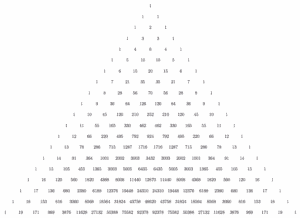Pythagorus was likely a huge inspiration to Blaise Pascal, famous french mathematician and philosopher who’s credited with coming up with “Pascal’s Triangle”. This triangle arises naturally in the exploration of combinatorics, and is build out of the binomial theorem meaning “two things added together”.

There are several interesting features of Pascal’s triangle that show the presence of a nested fractal harmony of information systems. It has the counting numbers in the first diagonal (not counting the row of 1s). It has the triangle numbers we find in the tetractys in diagonal line 2 who’s digital roots always sum to 3 6 9 or 1. In diagonal line 4 the distance between the sum of integers from the previous one and itself is always equal to 3 6 9 or 1. If you take any diagonal, any number of places and change directions, you’ll always get the sum of all the digits from the initial diagonal. If you take the sums from the horizontal rows you’ll get the powers of 2 or the doubling circuit. 1 2 4 8 16 32 64 128 and so on, or if you take the digital root 1 2 4 8 7 5 repeating all the way down.

Last but not least, if we take the shallow diagonals we get the fibonacci numbers.What if we kept going with the triangle numbers?

1+2+3+4+5+6+7+8+9+10+11= 66 = 3
1+2+3+4+5+6+7+8+9+10+11+12 = 78 = 6
1+2+3+4+5+6+7+8+9+10+11+12+13 = 91 =10
1+2+3+4+5+6+7+8+9+10+11+12+13+14 = 105 = 6
1+2+3+4+5+6+7+8+9+10+11+12+13+14+15 = 120 = 3
1+2+3+4+5+6+7+8+9+10+11+12+13+14+15+16 = 136 =10
1+2+3+4+5+6+7+8+9+10+11+12+13+14+15+16+17 = 153 = 9
1+2+3+4+5+6+7+8+9+10+11+12+13+14+15+16+17+18 = 171 = 9
1+2+3+4+5+6+7+8+9+10+11+12+13+14+15+16+17+18+19 = 190 = 10
1+2+3+4+5+6+7+8+9+10+11+12+13+14+15+16+17+18+19+20 = 210 = 3
1+2+3+4+5+6+7+8+9+10+11+12+13+14+15+16+17+18+19+20+21 = 231 = 6In Gematria – the exploration of the numeric properties of letters and words – Kabbalists are able to draw mathematically and geometrically consistent correspondences to language and meaning, giving them deeper insight into the hidden qualities of words and ideas. This is because it was built using this knowledge. The Hebrew language which is based on 22 flame letters all variations on the Yod, have been shown by the Meru research institute to emerge out of the golden ratio spiral on the tube torus. Its also been suggested that frequency vibrations on the Chladni plate showing cymatic vibrations contain the character forms of the flame letters, but to find all of them we’d need to see cymatic patterns in their 3d form. Chladini plates are 2d representation of what is actually higher dimensional.These 22 letters are made from the arithmetic and geometric symmetry that arises from the power of triangles. Its as if they are steganographicly and cryptographically encoded into the fundamental geometry of creation, making its language incredibly effective in maintaining isomorphic correlations and correspondences across four domains of reality. This means that the language of, let’s say chemistry and the language of geometry maintain a congruence of self similarity which we can track through this system.

We’ll look at this interesting feature another time, but for now I wanted to point out how the triangle numbers have particularly significant gematria correspondences.

The 5th triangle number is 15 = YH or YAH meaning “He is” which is 1/2 of the tetragramaton, and the divine name associated with the 2nd Sephira on the Tree of Life Chokmah meaning Wisdom.

The 6th triangle number is 21 = AHIH – Meaning “I AM” – The divine name associated with the 1st Sephirah Kether on the Tree of Life meaning Crown. 21 is the smallest non-trivial example of a Fibonacci number whose digits are Fibonacci numbers and whose digit sum is also a Fibonacci number.

The 7th triangle number is 28 = KH – Meaning Strength, or Capacity to attain. And YHDU – Meaning all together or UNION. 28 is the second perfect number. As a perfect number, it is related to the Mersenne prime 7, since 2(3 − 1)(23 − 1) = 28. The next perfect number is 496, the previous being 6. Twenty-eight is a harmonic divisor number, a happy number, a triangular number, a hexagonal number, and a centered nonagonal number. In the Torah it says “In the beginning God created the heavens and the earth.” In Hebrew, this verse has seven words and 28 (7 × 4) letters. The number seven is repeated throughout the Bible as a number of perfection and completeness. In Alchemy 7 encodes the rules of phase translation, or the ascent of the initiate from one level to the next in the completion of the great work.

The 8th triangle number 36 = AHL -Meaning “Eloha” — divine name associated with the 5th Sephira Geburah Meaning Strength or Courage. Kabbalists hold that the number 36 has special significance in the building of the universe: According to the Midrash, the light created by God on the first day of creation shone for exactly 36 hours; it was replaced by the light of the Sun that was created on the Fourth Day. The Torah commands 36 times to love, respect and protect the stranger. And, every generation it is said there are 36 righteous people (the “Lamed Vav Tzadikim”) in whose merit the world continues to exist. In the modern celebration of Hanukkah, 36 candles are kindled in the menorah over the eight days of that holiday (not including the shamash candle)

The 9th triangle number 45 = ADM “Adam” — the first man; man, men, mankind; a male.

the 10th triangle number 55 = HKL meaning ALL, Everything. KHL = Meaning consummation or end. “And God saw every thing that he had made, and, behold, it was very good. And the evening and the morning were the sixth day.” When ADM ascends through the 10 Sephirah creation is completed in the consummation of his being with Kether.

### How to build a universe

231 the 21st triangle number is a significant number from the Sepher Yetzirah. The Sepher Yetzirah, (Book of Creation) describes the formation of the universe through 22 Hebrew letters. In it the writer tells us to connect each pair of Hebrew letters in a circle. Each line connecting the pairs are called gates. We are told that meditating on each of the combinations of 22 pairs will give us insight into the nature of God.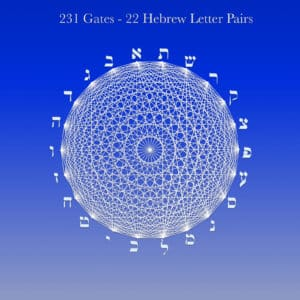There are 231 pairs of combinations. Remember Pascal’s triangle? In this form Pascal’s binomials are giving us an areal view of a tube torus. In this picture there’s no movement, but if we to animate it by applying the doubling circuit, it would begin to rotate.

If you look carefully you’ll see there is a series of 10 nested circles resulting from the overlapping lines showing us again that the Decad is present with the iterated tetrad, even as it is permuted into higher orders of complexity. Another significant fact worth mentioning, since we are arranging the 22 letters in a circle it would be appropriate to understand the reason for counting 360 degrees.

The number 60 has exactly 22 factors. 2, 3, 4, 5, 6, 8, 9, 10, 12, 15, 18, 20, 24, 30, 36, 40, 45, 60, 72, 90, 120, and 180. No other 2 digit number has such a massive entourage. This becomes important when considering why we have 360 degrees in a circle why we measure time in base 60 and why there are 12 signs of the zodiac and 12 notes in music.

It might not be obvious why numbers are intuitively transposable to hertz frequencies. One of the peculiar features of Source Vibrations music is that esoteric number theory is applied through out it. This is why. The number of oscillations of a wave form from peak to trough over time is a measure of cycles per second. To demonstrate why this works we need to understand how the division of time to units of 60 minutes and hour and seconds in a minute and the division of a circle into 360 degrees is exactly right.

60 has 22 factors, but 60 Also has 12 divisors. The largest number of divisors of any other 2 digit number. This makes itparticularly convenient for record keeping and making change which is why the ancient Babylonian and Sumerians used the 12 / 60 counting system as their preferred way of keeping measure. 1, 2, 3, 4, 5, 6, 10, 12, 15, 20, 30, 60. We’re still using it for our measure of time, our geometry and maps because there isn’t anything better. We can actually find this counting system embedded into our own physiology, suggesting its inherent in biological structures as well. Notice how on each of your four fingers you have 3 knuckle joints. Using your thumb you can count each of your joints to 12 on each hand.

6 X 60 is a perfect harmonic symmetry or fractal symmetry that exists at a high order of congruence. Remember that it takes 6 triangles edge to edge to complete a revolution of triangles. 360 / 12 = 30 or 3 x 10 – a Triad of Decads, just like our triangle numbers arising out of the tetractys.

Lets recap; we went from 3s and 4s to 10s noticing a vibrating 2 throughout. From the tertractys we saw the beginning of the quadternio, the four fold minor completion of being. We saw the fractal scaling of triangles embedding harmony wherever they go. We saw the golden ratio hiding in our still images as motion potential. We went on a tour du force of esoteric number theory, from arithmetic to geometry to music and beyond. But in order to really drive this experiment home. It needs to be as simple as can be expressed.

What does come into being? How ever we may define it, its term is 1. What can be said about it that is true? What must happen to it in order to create a number sequence. And what might the activity tell us about what the universe is. In the next section we will explore what it is that actually comes into being watching as it emerges from a singularity and unravels into time, form and multiplicity.